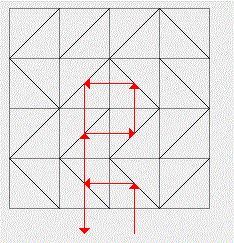# Marathon Match 80 - Marathon Match 80

## Key Information

Register
Submit
The challenge is finished.

## Challenge Overview

### Problem Statement

IMPORTANT: This problem is used for two simultaneous matches: TCO'13 Marathon Round 2 and Marathon Match 80. You can compete in TCO'13 R2 only if you are eligible for TCO'13 and haven't advanced from TCO'13 R1. You can compete in MM 80 if you are not eligible for TCO'13, have advanced from TCO'13 R1 or just would like to skip TCO'13 R2 by some reason. Competing in both matches is not allowed. Doing so will lead to disqualification. Note that registration does not count as competing. In order to be considered a competitor, you need to make at least one submit (example or full).

There is a square board separated into NxN square cells. Initially each cell contains a single mirror which is a segment that connects its two opposite corners. If a mirror connects top right and bottom left corners, it is denoted with letter 'L'. If it connects top left and bottom right corners, then it is denoted with 'R'.

The original state of the board will be given to you as a String[] board. Each element contains N characters and represents a single row. The characters are mirrors in that row listed left to right. The rows are given in the top to bottom order. Consider an example:Such board would be represented as {"LLLR", "RLRR", "LLLL", "RRRL"}.

By some reason you are not comfortable with all those mirrors and you want to destroy them. You've got a laser which can be used to cast rays. A ray is cast from outside of the board perpendicular to one of its side and so that it intersects (enters) this side exactly at the middle of some cell. The ray moves straight until it hits a mirror. Then it is reflected by physics laws and the mirror is instantly destroyed (even before the ray is able to exit the given cell). The process continues in this fashion and ends when the ray exits the board. Here is an example:Let's number the rows (top to bottom) and columns (left to right) with numbers 0 to N-1. Let's also assume there are imaginary rows -1 (above row 0) and N (below row N-1), as well as columns -1 (to the left of column 0) and N (to the right of column N-1). Then the ray follows the following rote in (row, column) coordinates: (4, 2) - (3, 2) - (3, 1) - (2, 1) - (2, 2) - (1, 2) - (1, 1) - (4, 1). Note that when the ray reaches cell (1, 1), the mirrors at (2, 1) and (3, 1) are already destroyed, so the ray will just exit the board after getting reflected at (1, 1).

Since your goal is to destroy all mirrors, you will continue casting rays until all of them are destroyed. For example, you can cast a second ray as follows:After that all mirrors are destroyed, so you're done.

The task is to achieve the goal using as small number of rays as possible. Your score will be equal to N/R (exact division), where R is the number of rays you used. Your overall score is the sum of scores on all test cases.

You will need to implement the method destroy. It takes board as the input in the format described above. The return value needs to be an int[] containing 2*R elements. The elements 2*i and 2*i+1 must represent the row and the column of the starting cell of the i-th ray. We assume that each ray starts in some imaginary row or column as in pictures above. Note that the order of elements in return value is important. For the example above, the return value would be {4, 2, -1, 1}.

The return value is allowed to contain at most 2*N*N elements, otherwise your score for the test case will be 0. It will also be 0 in case of other failures such as exceeding time or memory limit, returning result in invalid format or not being able to destroy all mirrors.

Test cases are generated as follows. N is selected uniformly, at random, between 50 and 100. Then each of the N*N cells is independently generated as 'L' with 50% probability and as 'R' otherwise.

An offline tester/visualizer is available.

### Definition

 Class: FragileMirrors Method: destroy Parameters: String[] Returns: int[] Method signature: int[] destroy(String[] board) (be sure your method is public)

### Notes

-The time limit is 10 seconds (this includes only the time spent in your code). The memory limit is 1024 megabytes.
-There is no explicit code size limit. The implicit source code size limit is around 1 MB (it is not advisable to submit codes of size close to that or larger). Once your code is compiled, the binary size should not exceed 1 MB.
-The compilation time limit is 30 seconds. You can find information about compilers that we use and compilation options here.
-There are 10 example test cases and 100 full submission (provisional) test cases. Example test cases use seeds 1 to 10.

### Examples

0)

 `"1"`
`Returns: "Seed = 1, N = 51"`
1)

 `"2"`
`Returns: "Seed = 2, N = 74"`
2)

 `"3"`
`Returns: "Seed = 3, N = 87"`
3)

 `"4"`
`Returns: "Seed = 4, N = 74"`
4)

 `"5"`
`Returns: "Seed = 5, N = 85"`
5)

 `"6"`
`Returns: "Seed = 6, N = 92"`
6)

 `"7"`
`Returns: "Seed = 7, N = 92"`
7)

 `"8"`
`Returns: "Seed = 8, N = 87"`
8)

 `"9"`
`Returns: "Seed = 9, N = 81"`
9)

 `"10"`
`Returns: "Seed = 10, N = 69"`

This problem statement is the exclusive and proprietary property of TopCoder, Inc. Any unauthorized use or reproduction of this information without the prior written consent of TopCoder, Inc. is strictly prohibited. (c)2020, TopCoder, Inc. All rights reserved.

## LEARN:

Topcoder Challenges Explained